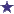# How many cc of 1:20 solution is needed to obtain a dose of 500mg? (w/v)

by Karina
(Bell)Pharmacy Ratio Question: Weight/Volume

How many cc of 1:20 solution is needed to obtain a dose of 500mg? (w/v)

### Comments for How many cc of 1:20 solution is needed to obtain a dose of 500mg? (w/v)

Average RatingRatingRemember to Covert The Units by: Anonymous Convert 1g to 1000mg The answer should be 10cc.

 RatingAnswer to Weight/Volume Question by: Jeremy 25cc's of a 1:20 solution is needed to obtain a dosage of 500mg (weight/volume)How did I get this answer? 1:20 = X:500Divide 500 by 20 and the answer is 25cc's (or ML, which is the same thing)